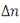# Drift and Diffusion Current - Engineering Physics

Drift and Diffusion

The current that flows across a semiconducting crystal has two components :

1. Drift current

2. Diffusion current

In a perfect crystal, the periodic electric field enables electrons and holes to move freely as if it in a vacuum. In this case, the wave model of the electron is more appropriate than the particle model. The defects in real crystals cause the periodic electric field to be distributed and for , thermal energy can be considered to cause the lattice ion to vibrate. These imperfection cause scattering of the free carriers resulting in a finite conductivity.

In the absence of any extremely applied electric field, the random motion of free carriers within a crystal does not result in a net transfer of charge since charge movement in any direction is balanced by charge movement in any other direction.

When a voltage is applied across the material, each carrier experiences a force attracting it to one end of the material. Electrons being attracted to the positive potential due to their negative charge and to the negative potential. This net movement in charge carriers termed drift is superimposed on the random thermal movement and result in current flow through the crystal. The electric current density is given by

If the material is a semiconductor, the current flow would be done electron and hole movement. Correspondingly the current densities due to electron drift and hole drift are

Respectively, these charge movement are in opposite directions, the electron movement is negative charge movement while the hole component is positive charge movement and so the total drift current is the sum of the two components, thus

i.e.

shows that the conductivity of a semiconductor is given by

For an intrinsic semiconductor, conductivity is given by

For extrinsic semiconductor with typical dopant levels, the minority carrier component is negligible at normal operating temperature.

Conductivity is a function of temperature. If an increase of temperature cause an increase in free charge, the conductivity odf the material is increased. However, temperature increase also cause increased vibration of the lattice which causes increased scattering, reduced carriers mobility and hence reduced conductivity. The variation of conductivity of a material with temperature depends on which of these effect dominates. For a conductor there is not a significant increase in free charge with increase of temperature and therefore the increased scattering causes the conductivity of a conductor to fall as the temperature increases. Alternatively, the free carrier density of a semiconductor increases rapidly with temperature resulting in increased conductivity. A conductor is said to have a positive temperature coefficient resistant since its resistance increases with temperature while a semiconductor has a negative coefficient.

At normal operating temperature an extrinsic semiconductor has properties similar to these of a conductor. An increase of temperature does not significantly change the free carrier density. Since most of the dopant atoms are already ionized, thus increased scattering causes conductivity to fall. However, as the transition temperature is approached, the generation of electron-hole pairs does cause the free carrier density to increase significantly and the material takes on intrinsic properties. Thus an extrinsic semiconductor has a positive temperature coefficient of resistance at normal temperature changing to a negative coefficient near the transition temperature.

Diffusion Current

In addition the drift motion of the carriers under the influence of an electric field the carriers in semiconductors may move by diffusion. Diffusion occurs whenever there is a non-uniform concentration of charge carriers at some places of the crystal. The free electron density in a homogeneous semiconductor in the absence of an applied electric field is uniform at equilibrium due to a combination of the random thermal motion of the electrons and the repulsive force between them caused by their like charge. If excess carrier are introduced locally within such a semiconductor, either by causing carrier generation by heating or incident radiation or by injecting carriers into the material via a surface contact, a non-uniform distribution is created. Ignoring the process of recombination which is an additional phenomenon, the excess carriers move from the region of higher density to regions of lower density tending to produce a uniform distribution. The transport mechanism is called diffusion and it take place in addition to drift caused by an applied electric field.

of electrons varies with distance x in the semiconductor, the concentration of gradient being

Partial derivatives are used here asis a function of both time and distance. Fick’s law states that the rate at which carriers diffuse is proportional to the density gradient and the movement is in the direction of negative gradient, the rate of flow of electrons is proportional to

From which the rate of flow across unit area is equal to

is the diffusion coefficient for electrons in the semiconductors concerned. This flow constitutes an electron diffusion current density and since conventional current is the rate of positive charge

If an excess hole concentration is created in the same region, hole diffusion takes place in the same direction at a rate per unit area